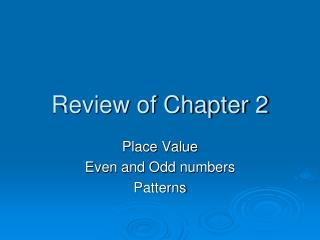DownloadDownload PresentationReview of Chapter 2

# Review of Chapter 2

Télécharger la présentation## Review of Chapter 2

- - - - - - - - - - - - - - - - - - - - - - - - - - - E N D - - - - - - - - - - - - - - - - - - - - - - - - - - -
##### Presentation Transcript

1. Review of Chapter 2 Place Value Even and Odd numbers Patterns

2. 346 - 40

3. Write the following number in standard form. 10,000 + 6,000 + 900 + 60 + 5

4. 5,487 5,000 + 400 + 80 + 7

5. Predict the next number in the pattern. 310, 410, 510, 610, ______

6. 310, 410, 510, 610, 710 We have a pattern of adding 100 each time.

7. 536,173 500,000 + 30,00 + 6,000 + 100 + 70 + 3

8. 123,789 100,000

9. Write the following number in standard form. Twenty-two thousand, eighteen

10. Katie made a model using base ten blocks. She used 4 hundreds, 12 tens, and 8 ones. What number did she make?

11. She used 4 hundreds, 12 tens, and 8 ones. 4 hundreds = 400 12 tens = 120 8 ones = 8 400 + 120 + 8 = 528

12. Predict the next two numbers in the pattern. 508, 512, 510, 514, 512, ____, ____

13. 508, 512, 510, 514, 512, 516, 514 • We have a pattern of adding 4 then subtracting 2.

14. Write the following number in standard form. 600,000 + 50,000 + 3,000 + 5

15. 600,000 + 50,000 +3,000 + 5 = 653, 005

16. 929 - odd

17. Ray modeled the number 137 with base ten blocks. He used 1 hundred and 2 tens. How many ones blocks did he use?

18. The number modeled was 137. He used 1 hundred and 2 tens. 1 hundred = 100 2 tens = 20 100 + 20 = 120 137 – 120 = 17 ones blocks

19. 376,055 300,000 + 70,000 + 6,000 + 50 + 5

20. 348 - even

21. Write the following number in standard form. Two thousand, four hundred sixty-seven

22. 458,322 50,000